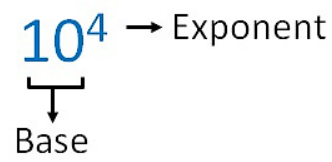# exponents and powers

## EXPONENTIATION:

Linear Equations in One VExponentiation is a mathematical operation, written as bn, involving two numbers, the base b and the exponent (or power) n. When n is a positive integer, exponentiation corresponds to repeated multiplication; in other words, a product of n factors, each of which is equal to b (the product itself can also be called power):ariable

bn = b0 + b1 + b2 + b3............bn

E.g. -The exponent is usually shown as a superscript to the right of the base.